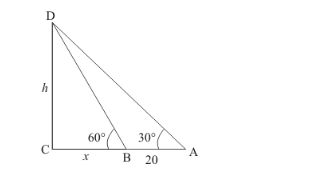# The angle of elevation of the top of a tower from a point A on the ground is 30°.

Question:

The angle of elevation of the top of a tower from a point A on the ground is 30°. On moving a distance of 20 metres towards the foot of the tower to a point B the angle of elevation increases to 60°. Find the height of the tower and the distance of the tower from the point A.

Solution:

Letbe height of tower and the angle of elevation of the top of tower from a pointon the ground isand on moving with distancem towards the foot of tower on the pointis.

LetandNow we have to find height of tower and distance of tower from point A.

So we use trigonometrical ratios.In,

$\Rightarrow \quad \tan D=\frac{C D}{B C}$

$\Rightarrow \quad \tan 60^{\circ}=\frac{C D}{B C}$

$\Rightarrow \quad \sqrt{3}=\frac{h}{x}$

$\Rightarrow \quad x=\frac{h}{\sqrt{3}}$

Again in,

$\Rightarrow \quad \tan A=\frac{C D}{B C+B A}$

$\Rightarrow \quad \tan 30^{\circ}=\frac{h}{x+20}$

$\Rightarrow \quad \frac{1}{\sqrt{3}}=\frac{h}{x+20}$

$\Rightarrow \quad x=\sqrt{3} h-20$

$\Rightarrow \frac{h}{\sqrt{3}}+20=\sqrt{3} h$

$\Rightarrow \frac{h}{\sqrt{3}}-\sqrt{3} h=-20$

$\Rightarrow \quad h-3 h=-20 \sqrt{3}$

$\Rightarrow \quad-2 h=-20 \sqrt{3}$

$\Rightarrow \quad h=10 \sqrt{3}$

$\Rightarrow \quad h=17.32$

$\Rightarrow \quad x=\frac{10 \sqrt{3}}{\sqrt{3}}$

$\Rightarrow \quad x=10$

So distance

$\Rightarrow \quad A C=x+20$

$\Rightarrow \quad A C=30$

Hence the required height is $17.32 \mathrm{~m}$ and distance is $30 \mathrm{~m}$.